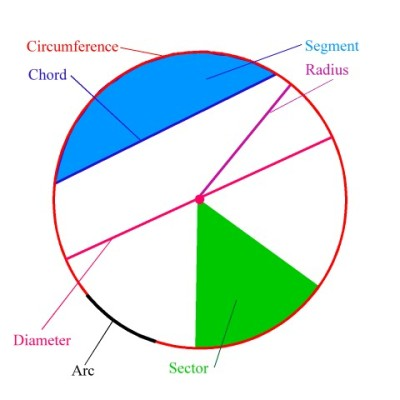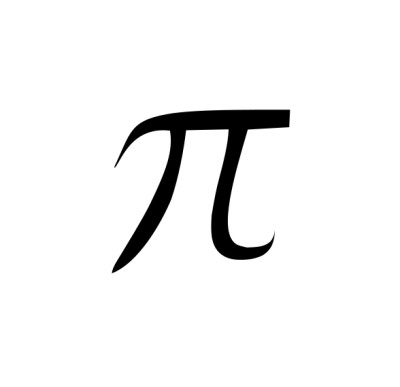List of public pages created with Protopage

# Home

## Plain sticky notes

### Indices!

1n= 1 n1= n n0= 1 naxnb= na+b na÷nb n1/2=√n n1/3=3√n

### Number sequence! (Nth Term)

2, 5, 8, 11, 14, Rule- 2n+3

### Fractions, decimals & percentages!

Decimal-Percentage= x100 Percentage-Decimal= ÷100 Percentage-Fraction= /100 then simplify Fraction-Decimal= Numerator÷Denominator Decimal-Fraction= Look at what column the last digit is in then make into fraction over this e.g. 10/100/1000

### Square roots!

1= 1 2= 4 3= 9 4= 16 5= 25 6= 36 7= 49 8= 64 9= 81 10= 100 11= 121 12= 144

### Types of angles!

Z angle- Alternate C angle- Supplementry F angle- Corresponding

### y=mx+c

Gradient= m (steepness) y-intercept= c (crosses the y axis)

## Photos

### Parts of a Circle!### Pi!# Bookmarks

## Plain sticky notes

### Sticky note

Click 'edit' on the Bookmarks widget to add all of your favorite sites to your page for quick access

# Notes

## Plain sticky notes

### Sticky note

Click this text to change it...